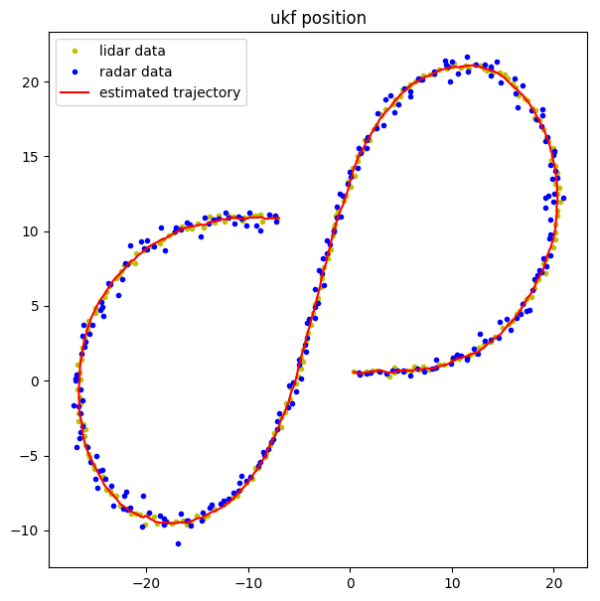## The project

I try to avoid publishing my code solving homework assignments, but this Udacity SDC project is generic enough to be useful in a wider context. So here you have it. The task was to fuse together radar and lidar measurements using two kinds of Kalman Filters to estimate the trajectory of a moving bicycle. The unscented filter uses the CTRV model tracking the position, speed, yaw, and yaw rate, whereas the extended filter uses the constant velocity model.The Unscented Filter result

Both algorithms performed well, with the CTRV model predicting the velocity significantly better. The values below are RMSEs of the prediction against the ground truth. The first two values represent the position, the last two - the velocity.

Extended filter:

``````]==>  ./ExtendedKF  ../data/obj_pose-laser-radar-synthetic-input.txt ../src/ekf.txt
Accuracy - RMSE:
0.0973826
0.0853209
0.441738
0.453757
``````

Unscented filter:

``````]==>  ./UnscentedKF  ../data/obj_pose-laser-radar-synthetic-input.txt ../src/ukf.txt
Accuracy - RMSE:
0.0659867
0.0811041
0.277747
0.166186
``````

## The code

I wrote a handy library that does most of the math and provides various concrete implementations of Kalman predictors and updaters:

``` 1class KalmanPredictor {
2  public:
3    virtual ~KalmanPredictor() {}
4    virtual void Predict(KalmanState &state, uint64_t dt) = 0;
5};
6
7class KalmanUpdater {
8  public:
9    virtual ~KalmanUpdater() {}
10    virtual void Update(KalmanState           &state,
11                        const Eigen::VectorXd &z) = 0;
12};
```

The code you need to implement yourself depends on the sensor, the model, and the type of the filter you use. Ie., for the CTRV model and a Lidar measurement you only need to specify the projection matrix and the sensor noise covariance:

``` 1class LidarUpdater: public LinearKalmanUpdater {
2  public:
3    LidarUpdater() {
4      H_ = MatrixXd(2, 5);
5      H_ << 1, 0, 0, 0, 0,
6            0, 1, 0, 0, 0;
7
8      R_ = MatrixXd(2, 2);
9      R_ << 0.0225,      0,
10            0,      0.0225;
11    }
12};
```

See here and here for more examples. The state travels around in an object of the KalmanState class:

``` 1struct KalmanState {
2  KalmanState(int n) {
3    x = Eigen::VectorXd(n);
4    P = Eigen::MatrixXd(n, n);
5    x.fill(0.0);
6    P.fill(0.0);
7  }
8  Eigen::VectorXd x;              // mean
9  Eigen::MatrixXd P;              // covariance
10  Eigen::MatrixXd sigma_points;   // sigma points
11  double          nis = 0;        // Normalized Innovation Squared
12};
```

All this ends up with the measurement update code boiling down to this:

```1double dt = measurement.timestamp - previous_timestamp_;
2previous_timestamp_ = measurement.timestamp;
3predictor_->Predict(state_, dt);
4updaters_[measurement.sensor_type]->Update(state_, measurement.data);
5return state_;
```

See the full code on GitHub.

If you like this kind of content, you can subscribe to my newsletter, follow me on Twitter, or subscribe to my RSS channel.